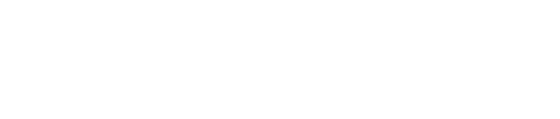01/01/2003

# Does Deregulation Change Economic Behavior of Firms?

Views: 1922
Cost minimization and profit maximization behavioral assumptions are most widely used in microeconomic theory to analyze firm behavior. However, in practice researchers do not know whether every firm in the sample maximizes profit or minimizes cost. In this paper we address this problem via a latent class modeling approach in which we first consider the cost minimization problem (first class) and then the profit maximization problem (second class). The two problems are then mixed and the probabilities of class membership are made functions of covariates. This approach does not require researchers to know which firms maximize profit and which ones minimize cost. On the contrary, it helps us to determine not only which firms behave like profit maximizers but also why and what differentiates them from firms that failed to maximize profit. The new technique is illustrated using a panel data for the US airlines. The empirical findings suggest that very few airlines maximize profit consistently (if at all) and that deregulation had a positive impact on the chances of behaving like profit maximizers, although very few airlines continue to maximize profit even after the deregulation.